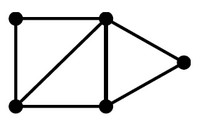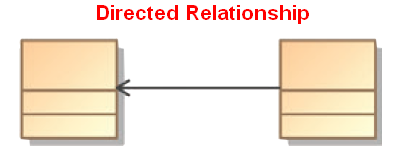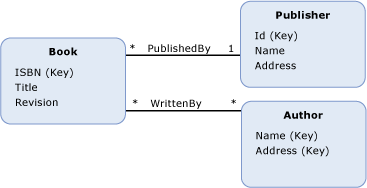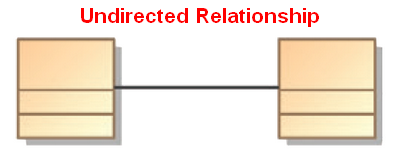# Logical Data Modeling - Asymmetric Relation (Uni-directional|Antisymmetric)

An asymmetric relation is a type of binary relation that requiers:

• antisymmetry (ie if a is related to b, b is not related to a)
• and irreflexivity (ie an element cannot be related to itself)

Antisymmetry is different from asymmetry because it does not requier irreflexivity, therefore every asymmetric relation is antisymmetric, but the reverse is false.

A relation that is not asymmetric, is symmetric.

A asymmetric relation is an directed relationship .

It's also known as a uni-directional relationship.

## Representation

### Entity Model

When there is a direction on the association, the relationship is asymmetric.

### Set of tuple

A asymmetric relation in the set of {1,2,3} would be the set of tuple

``````<1,3>
<2,1>
<3,2>
```
```

## Documentation / Reference

Discover More(Network|Graph) - Directed Graph (or digraph)

A directed graph (or digraph) is a graph, where the edges have a direction associated with them. Directed edges are suitable for modeling asymmetric relations undirected Data flow modeling ...Graph - Direction

A direction is a property of an edge. Directed edges are suitable for modeling asymmetric relations Undirected edges are suitable for modeling symmetric relations An edge is directed if it has a...
Logical Data Modeling - Aggregation (Collection)

aggregation is a containment relationship where one or more entities are part of a container entity. In other word, aggregation is combining multiple pieces of data into one unit. The container entity...Logical Data Modeling - Antisymmetry relationship

A Antisymmetric relation is a relationship that happens when for all a and b in X: if a is related to b then b isNOT related to a or b=a (reflexivity is allowed) In mathematical notation, an Antisymmetric...Logical Data Modeling - Association

An association is a relationship between two or more entities. It's represented by a link in the data model. An association: answers to the question why two entity need to know one another. specifies...Logical Data Modeling - Binary Relation

A binary relation is a relationship between two elements that is implemented via a binary function. Binary relations are used in many branches of mathematics to model concepts like: order relation...Logical Data Modeling - Relationship

A relationship is a model element that relates two entities . In its simple form (semantic form), it's a description that has a verb such as: has consists of uses ... entityentity setrelationship...Logical Data Modeling - Relationship Direction (Directed/Undirected)

The direction of a relationship shows if the relationship can be interpreted in one or both directions. There is only two options: - an asymmetric relation - a symmetric relation. A directed...Logical Data Modeling - Symmetric relationship (bi-directional)

A symmetric relation is a type of binary relation that happens when for all a and b in X if a is related to b, then b is related to a In mathematical notation, the relation f between x and y is...Logical Data Modeling - Transitive Relationship Property

transitive is a relationship property that tells that the relationship follows the following rule: when the relation relates a to b and b to c, then the relation relates a to c. In mathematical notation,...